# ggplot2: layer by layer plotting

``````## knitr configuration: http://yihui.name/knitr/options#chunk_options
opts_chunk\$set(comment = "", error= TRUE, warning = FALSE, message = FALSE,
tidy = FALSE, cache = F, echo = T,
fig.width = 6, fig.height = 6)

## R configuration
options(width = 116, scipen = 5)
``````

## References

``````library(ggplot2)
data(diamonds)
``````

## Provide data and mapping

``````p <- ggplot(data = diamonds, mapping = aes(x = carat, y = price, color = cut))
summary(p)
``````
``````data: carat, cut, color, clarity, depth, table, price, x, y, z [53940x10]
mapping:  x = carat, y = price, colour = cut
faceting: facet_null()
``````

## layer function and its arguments

``````layer(geom, geom_params, stat, stat_params, data, mapping, position)
``````

## Add a layer with point geom

``````p + layer(geom = "point")
``````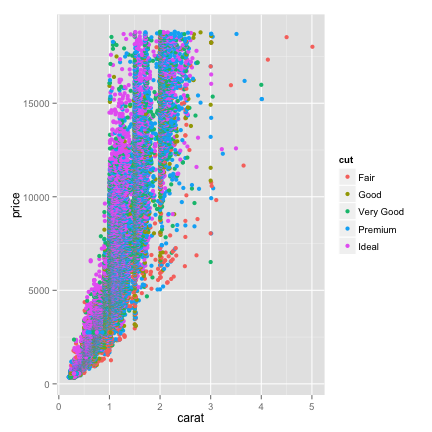point geom comes with identity stat and identitiy position

``````geom_point(mapping = NULL, data = NULL,
stat = "identity", position = "identity",
na.rm = FALSE, ...)
``````

More explicit expression

``````p + layer(geom = "point", stat = "identity", position = "identity")
``````

Abbreviated expression

``````p + geom_point()
``````

## Add a layer with bar geom

Map carat to x axis for histogram.

``````p <- ggplot(data = diamonds, mapping = aes(x = carat))
summary(p)
``````
``````data: carat, cut, color, clarity, depth, table, price, x, y, z [53940x10]
mapping:  x = carat
faceting: facet_null()
``````

bar geom and histogram geom come with bin stat and stack position by default

``````geom_bar(mapping = NULL, data = NULL, stat = "bin",
position = "stack", ...)

geom_histogram(mapping = NULL, data = NULL, stat = "bin",
position = "stack", ...)
``````

Create histogram explicitly

``````p2 <- p + layer(
geom = "bar",
geom_params = list(fill = "white", color = "steelblue"),
stat = "bin",
stat_params = list(binwidth = 0.5)
)
summary(p2)
``````
``````data: carat, cut, color, clarity, depth, table, price, x, y, z [53940x10]
mapping:  x = carat
faceting: facet_null()
-----------------------------------
geom_bar: fill = white, colour = steelblue
stat_bin: binwidth = 0.5
position_stack: (width = NULL, height = NULL)
``````
``````p2
``````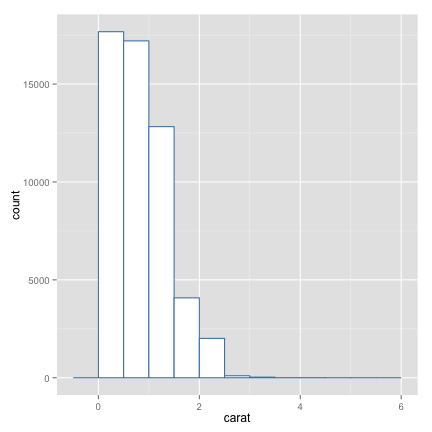Another way of doing the same

``````p + layer(
geom = "histogram",
geom_params = list(fill = "white", color = "steelblue"),
stat = "bin",
stat_params = list(binwidth = 0.5)
)
``````

## Add another layer of smoothing

``````data(msleep)
``````
``````                        name      genus  vore        order conservation sleep_total sleep_rem sleep_cycle awake
1                    Cheetah   Acinonyx carni    Carnivora           lc        12.1        NA          NA  11.9
2                 Owl monkey      Aotus  omni     Primates         <NA>        17.0       1.8          NA   7.0
3            Mountain beaver Aplodontia herbi     Rodentia           nt        14.4       2.4          NA   9.6
4 Greater short-tailed shrew    Blarina  omni Soricomorpha           lc        14.9       2.3      0.1333   9.1
5                        Cow        Bos herbi Artiodactyla domesticated         4.0       0.7      0.6667  20.0
6           Three-toed sloth   Bradypus herbi       Pilosa         <NA>        14.4       2.2      0.7667   9.6
brainwt  bodywt
1      NA  50.000
2 0.01550   0.480
3      NA   1.350
4 0.00029   0.019
5 0.42300 600.000
6      NA   3.850
``````

Map REM sleep proportion to x axis, and awake time to y axis

``````p <- ggplot(data = msleep, mapping = aes(x = sleep_rem / sleep_total, y = awake))
summary(p)
``````
``````data: name, genus, vore, order, conservation, sleep_total, sleep_rem, sleep_cycle, awake, brainwt,
bodywt [83x11]
mapping:  x = sleep_rem/sleep_total, y = awake
faceting: facet_null()
``````

smooth geom comes with smooth stat and vice versa

``````geom_smooth(mapping = NULL, data = NULL, stat = "smooth",
position = "identity", ...)

stat_smooth(mapping = NULL, data = NULL, geom = "smooth",
position = "identity", method = "auto",
formula = y ~ x, se = TRUE, n = 80, fullrange = FALSE,
level = 0.95, na.rm = FALSE, ...)
``````

Thus, adding geom = “smooth” and stat = “smooth” are the same

``````p3.a <- p + layer(geom = "point") + layer(geom = "smooth")
p3.b <- p + layer(geom = "point") + layer(stat = "smooth")
summary(p3.a)
``````
``````data: name, genus, vore, order, conservation, sleep_total, sleep_rem, sleep_cycle, awake, brainwt,
bodywt [83x11]
mapping:  x = sleep_rem/sleep_total, y = awake
faceting: facet_null()
-----------------------------------
geom_point:
stat_identity:
position_identity: (width = NULL, height = NULL)

geom_smooth:
stat_smooth:
position_identity: (width = NULL, height = NULL)
``````
``````p3.a
``````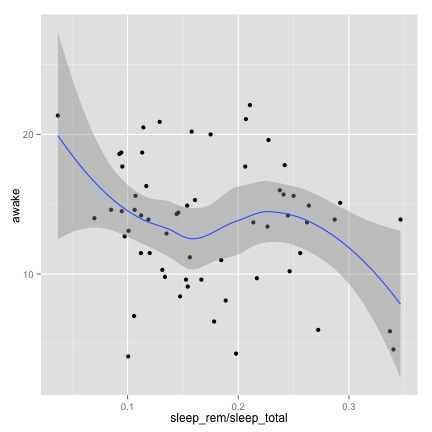Give additional arguments to smooth stat via stat_param

``````p + layer(geom = "point") +
layer(stat = "smooth", stat_params = list(method = "lm", se = F))
``````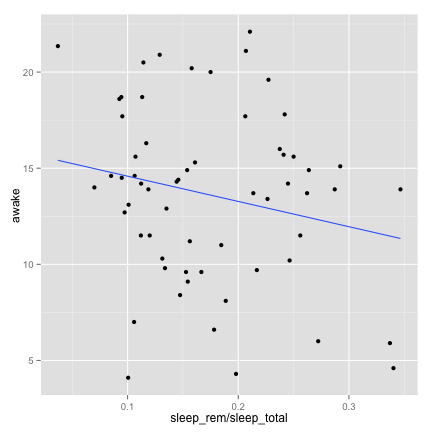Give more additional arguments to smooth geom via geom_params

``````p + layer(geom = "point") +
layer(geom = "smooth",
geom_params = list(color = "red", alpha = 0.5),
stat = "smooth",
stat_params = list(method = "lm", se = F)
)
``````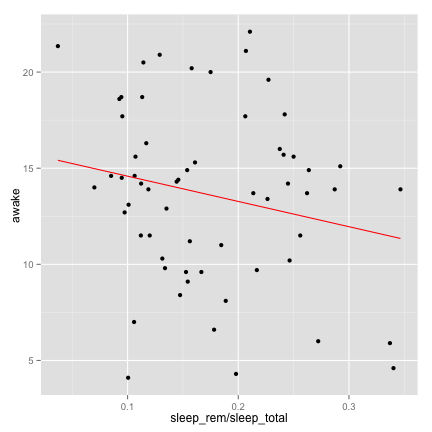A layer can be stored in an object and recycled

``````smoother <- geom_smooth(color = "red", alpha = 0.5, method = "lm", se = F)

p4 <- p + geom_point() + smoother
p4
````````````
ggplot(data = diamonds, mapping = aes(x = carat, y = price, color = cut)) + geom_point() + smoother
``````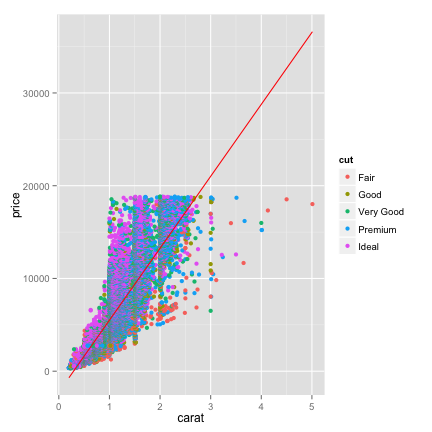The whole structure can be recycled and used with a different data frame

``````p4
````````````p4 %+% msleep[1:30,]
``````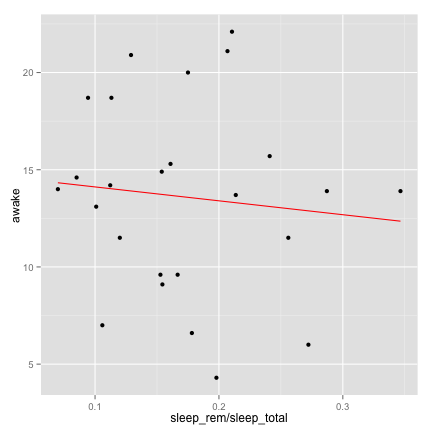## Add mapping after ggplot object

``````     ‘aes’ creates a list of unevaluated expressions.  This function
also performs partial name matching, converts color to colour, and
old style R names to ggplot names (eg. pch to shape, cex to size)
``````

Create ggplot object without mapping and then add mapping with aes()

``````data(mtcars)
p.mtcars <- ggplot(data = mtcars)
summary(p.mtcars)
``````
``````data: mpg, cyl, disp, hp, drat, wt, qsec, vs, am, gear, carb [32x11]
faceting: facet_null()
``````
``````p.mtcars <- p.mtcars + aes(x = wt, y = hp)
summary(p.mtcars)
``````
``````data: mpg, cyl, disp, hp, drat, wt, qsec, vs, am, gear, carb [32x11]
mapping:  x = wt, y = hp
faceting: facet_null()
``````

``````p.mtcars <- p.mtcars + layer(geom = "point", mapping = aes(color = factor(cyl)))
summary(p.mtcars)
``````
``````data: mpg, cyl, disp, hp, drat, wt, qsec, vs, am, gear, carb [32x11]
mapping:  x = wt, y = hp
faceting: facet_null()
-----------------------------------
mapping: colour = factor(cyl)
geom_point:
stat_identity:
position_identity: (width = NULL, height = NULL)
``````

Remove mapping by using NULL

``````p.mtcars <- p.mtcars + aes(y = NULL)
summary(p.mtcars)
``````
``````data: mpg, cyl, disp, hp, drat, wt, qsec, vs, am, gear, carb [32x11]
mapping:  y = NULL, x = wt
faceting: facet_null()
-----------------------------------
mapping: colour = factor(cyl)
geom_point:
stat_identity:
position_identity: (width = NULL, height = NULL)
``````

## Set to a fixed value and map to a variable

``````p.mtcars <- ggplot(data = mtcars, mapping = aes(x = mpg, y = wt))
summary(p.mtcars)
``````
``````data: mpg, cyl, disp, hp, drat, wt, qsec, vs, am, gear, carb [32x11]
mapping:  x = mpg, y = wt
faceting: facet_null()
``````
``````p.mtcars + geom_point()
``````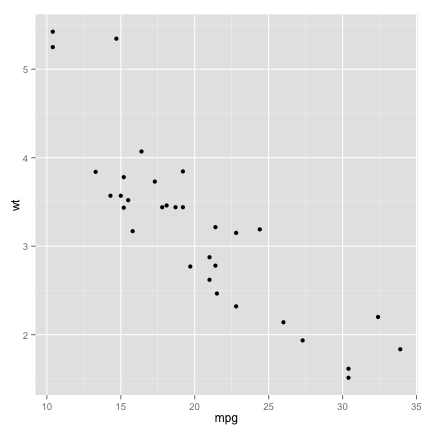Setting color to darkblue (correct)

``````p.mtcars + geom_point(color = "darkblue")
``````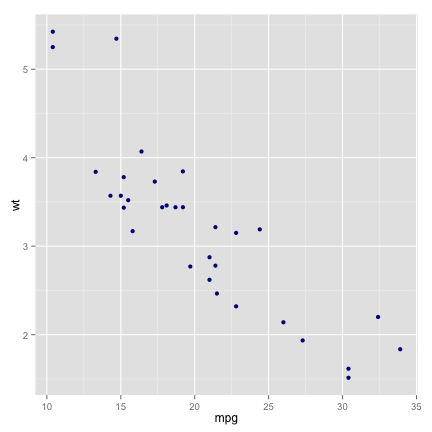Mapping color to a categorical variable with value “darkblue” (wrong)

``````p.mtcars + geom_point(mapping = aes(color = "darkblue"))
``````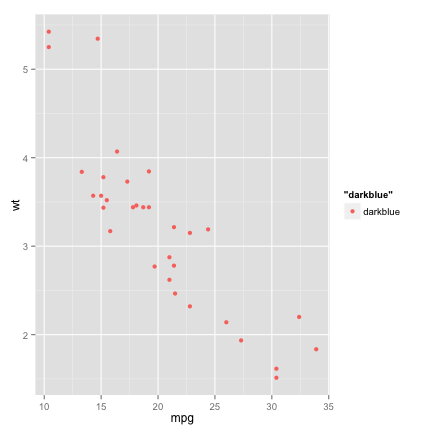## Group data points

``````library(nlme)
data(Oxboys)
``````
``````Grouped Data: height ~ age | Subject
Subject     age height Occasion
1       1 -1.0000  140.5        1
2       1 -0.7479  143.4        2
3       1 -0.4630  144.8        3
4       1 -0.1643  147.1        4
5       1 -0.0027  147.7        5
6       1  0.2466  150.2        6
``````
``````p.ox <- ggplot(data = Oxboys, mapping = aes(x = age, y = height, group = Subject))
summary(p.ox)
``````
``````data: Subject, age, height, Occasion [234x4]
mapping:  x = age, y = height, group = Subject
faceting: facet_null()
``````

Plot with appropriate grouping

``````p.ox <- p.ox + layer(geom = "line")
p.ox
``````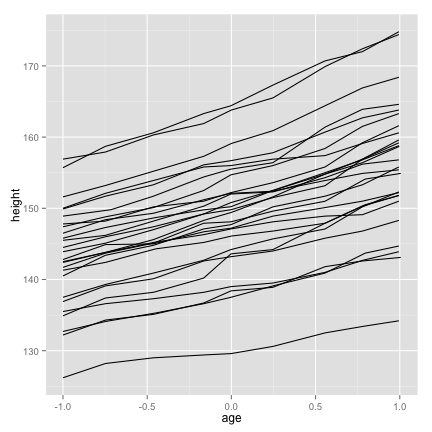Plot without grouping

``````p.ox + aes(group = NULL)
``````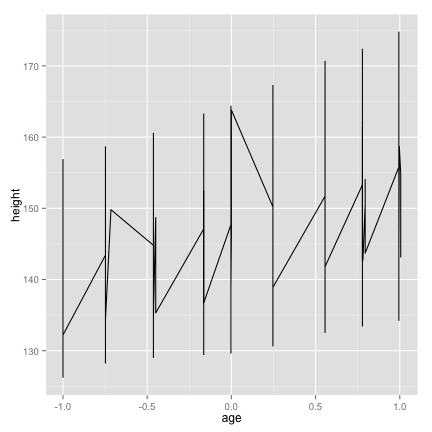Subject level grouping for line but no grouping for smooth

``````p.ox +
layer(geom = "smooth",
geom_params = list(lwd = 3),
stat_params = list(se = F, method = "lm"),
mapping = aes(group = NULL))
``````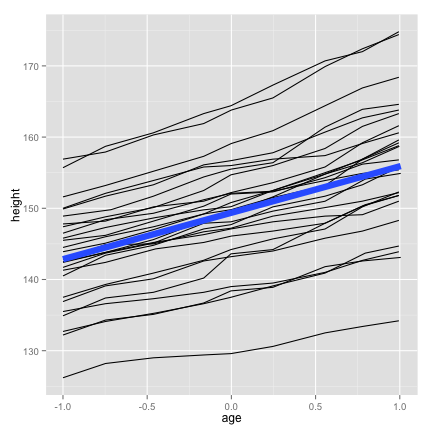## Use of boxplot

``````geom_boxplot(mapping = NULL, data = NULL,
stat = "boxplot", position = "dodge",
outlier.colour = "black", outlier.shape = 16,
outlier.size = 2, notch = FALSE, notchwidth = 0.5, ...)

stat_boxplot(mapping = NULL, data = NULL,
geom = "boxplot", position = "dodge", na.rm = FALSE,
coef = 1.5, ...)
``````
``````p.ox2 <- ggplot(data = Oxboys, mapping = aes(x = Occasion, y = height))
summary(p.ox2)
``````
``````data: Subject, age, height, Occasion [234x4]
mapping:  x = Occasion, y = height
faceting: facet_null()
``````
``````
p.ox3 <- p.ox2 + geom_boxplot() + geom_line(mapping = aes(group = Subject), color = "#3366FF")
summary(p.ox3)
``````
``````data: Subject, age, height, Occasion [234x4]
mapping:  x = Occasion, y = height
faceting: facet_null()
-----------------------------------
geom_boxplot: outlier.colour = black, outlier.shape = 16, outlier.size = 2, notch = FALSE, notchwidth = 0.5
stat_boxplot:
position_dodge: (width = NULL, height = NULL)

mapping: group = Subject
geom_line: colour = #3366FF
stat_identity:
position_identity: (width = NULL, height = NULL)
``````
``````p.ox3
``````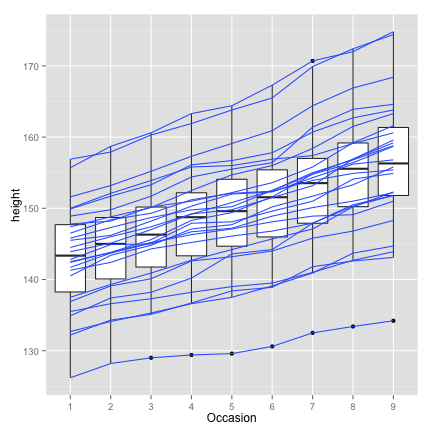## Use of summary stat

``````     ‘stat_summary’ allows for tremendous flexibilty in the
specification of summary functions.  The summary function can
either operate on a data frame (with argument name ‘fun.data’) or
on a vector (‘fun.y’, ‘fun.ymax’, ‘fun.ymin’).
``````
``````stat_summary(mapping = NULL, data = NULL,
geom = "pointrange", position = "identity", ...)
``````

summary stat and line geom do not work together (bug?)

``````p.ox3 + layer(geom = "line",
geom_params = list(color = "red", mapping = aes(group = 1)),
stat = "summary",
stat_params = list(fun.y = mean))
``````
``````geom_path: Each group consist of only one observation. Do you need to adjust the group aesthetic?
``````Create summary statistics data frame and override data option

``````library(plyr)
mean.at.occasions <-
ddply(.data = Oxboys, .variables = "Occasion",
.fun = summarize, mean.age = mean(age), mean.height = mean(height))

p.ox3 + layer(data = mean.at.occasions,
geom = "line",
geom_params = list(color = "red", size = 2),
stat = "identity",
mapping = aes(y = mean.height, group = 1))
``````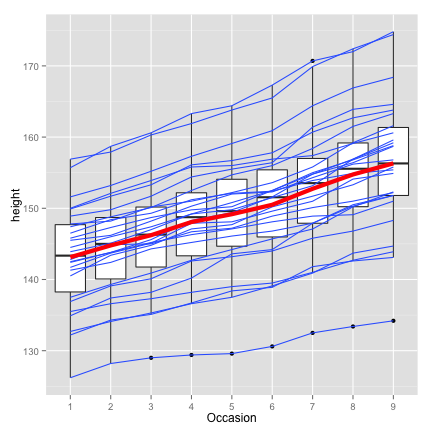Further summary statistics outside ggplot2

Red lines for min, mean, and max

``````height.at.occasions <-
ddply(.data = Oxboys, .variables = "Occasion",
.fun = summarize,
mean.height = mean(height),
min.height = min(height),
max.height = max(height))

library(reshape2)
height.at.occasions.melt <- melt(height.at.occasions)
p.ox3 + layer(data = height.at.occasions.melt,
geom = "line",
geom_params = list(color = "red", size = 2),
stat = "identity",
mapping = aes(y = value, group = variable))
``````